# The Boltzmann constant

The Boltzmann constant (k or kB) is the physical constant relating temperature to energy. It is named after the Austrian physicist Ludwig Eduard Boltzmann.

## Introduction

Its experimentally determined value (in SI units, 2002 CODATA value) is: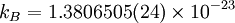$k_B =1.380 6505(24) \times 10^{-23}$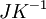$\left. JK^{-1}\right.$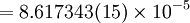$=8.617 343(15) \times 10^{-5}$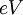$\left.eV\right.$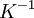$\left.K^{-1}\right.$

## History of Boltzmann's constant

"This constant is often referred to as Boltzmann's constant, although, to my knowledge, Boltzmann himself never introduced it - a peculiar state of affairs, which can be explained by the fact that Boltzmann, as appears from his occasional utterances, never gave thought to the possibility of carrying out an exact measurement of the constant."

Max Planck, Nobel Lecture, June 2, 1920

## Experimental determination of Boltzmann's constant

Boltzmann's constant can be obtained from the ratio of the molar gas constant to the Avogadro constant. The molar gas constant can be obtained via acoustic gas thermometry, and Avogadros constant from either the Silicon sphere, or via the watt balance. Recently laser spectroscopy has been used to determine the constant (Refs. 3 and 4). Other techniques include Coulomb blockade thermometry (Refs. 5 and 6).

1. L. Storm "Precision Measurements of the Boltzmann Constant",Metrologia 22 pp. 229-234 (1986)
2. B Fellmuth, Ch Gaiser and J Fischer "Determination of the Boltzmann constant—status and prospects", Measurement Science and Technology 17 pp. R145-R159 (2006)
3. C. Daussy, M. Guinet, A. Amy-Klein, K. Djerroud, Y. Hermier, S. Briaudeau, Ch. J. Bordé, and C. Chardonnet "Direct Determination of the Boltzmann Constant by an Optical Method", Physical Review Letters 98 250801 (2007)
4. G. Casa, A. Castrillo, G. Galzerano, R. Wehr, A. Merlone, D. Di Serafino, P. Laporta, and L. Gianfrani "Primary Gas Thermometry by Means of Laser-Absorption Spectroscopy: Determination of the Boltzmann Constant", Physical Review Letters 100 200801 (2008)
5. J. P. Pekola, K. P. Hirvi, J. P. Kauppinen, and M. A. Paalanen "Thermometry by Arrays of Tunnel Junctions", Physical Review Letters 73 pp. 2903-2906 (1994)
6. Jukka P. Pekola, Tommy Holmqvist, and Matthias Meschke "Primary Tunnel Junction Thermometry", Physical Review Letters 101 206801 (2008)

• SklogWiki